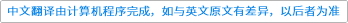Constructive Approximation ( IF 1.531 ) Pub Date : 2021-04-07 , DOI: 10.1007/s00365-021-09536-3
Baobin Wang, Ting Hu

Gaussians are a family of Mercer kernels which are widely used in machine learning and statistics. The variance of a Gaussian kernel reflexes the specific structure of the reproducing kernel Hilbert spaces (RKHS) induced by the Gaussian or other important features of learning problems such as the frequency of function components. As the variance of the Gaussian decreases, the learning performance and approximation ability will improve. This paper introduces the unregularized online algorithm with decreasing Gaussians where no regularization term is imposed and the samples are presented in sequence. With the appropriate step sizes, concrete learning rates are derived under the smoothness assumptions on the target function, for which are used to bound the approximation error. Additionally, a new type of the geometric noise condition is proposed to estimate the approximation error instead of any smoothness assumption. It is more general than the work in Steinwart et al. (Ann Stat 35(2):575–607, 2007), for which is only suitable for the hinge loss. An essential estimate is to bound the difference of the approximation functions generated by varying Gaussian RKHS. Fourier transform plays a crucial role in our analysis.down
wechat
bug